SLIS178B October   2017  – January 2018

PRODUCTION DATA.

1. Features
2. Applications
3. Description
4. Revision History
5. Description (Continued)
6. Pin Configuration and Functions
7. Specifications
8. Detailed Description
1. 8.1 Overview
2. 8.2 Functional Block Diagram
3. 8.3 Feature Description
1. 8.3.1 Device Bias
2. 8.3.2 Charge Pump
3. 8.3.3 Constant-Current Driving
4. 8.3.4 PWM Dimming
5. 8.3.5 Analog Dimming
6. 8.3.6 Output Current Derating
7. 8.3.7 Diagnostics and Fault
4. 8.4 Device Functional Modes
9. Application and Implementation
1. 9.1 Application Information
2. 9.2 Typical Applications
1. 9.2.1 Typical Application for Automotive Exterior Lighting With One-Fails–All-Fail
2. 9.2.2 High-Precision Dual-Brightness PWM Generation
3. 9.2.3 Driving High-Current LEDs With Parallel MOSFETs
10. 10Layout
11. 11Device and Documentation Support
12. 12Mechanical, Packaging, and Orderable Information

• PW|28
• PW|28

### 7.7 Typical Characteristics

TA = 25 ºC unless otherwise notedV(IN) = 14 V V(ICTRL) = 1.8 V
Figure 2. Full-Range Current-Sense Voltage vs Ambient Temperature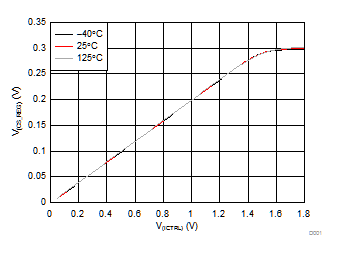V(IN) = 14 V
Figure 4. Current-Sense Voltage vs ICTRL Input Voltage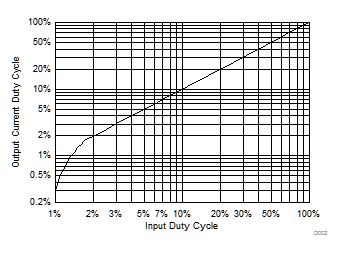V(IN) = 14 V f(PWM)= 200 Hz V(ICTRL) = 1.8 V
Figure 6. Duty Cycle of PWM Dimming-Current Output vs PWM Input Duty Cycle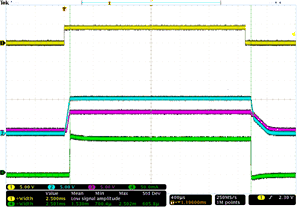Ch. 1 = PWM1 Ch. 2 = G1 Ch. 3 = SENSE1 Ch. 4 = I(OUT1) V(IN) = 14 V
Figure 8. PWM DimmingCh. 1 = V(IN) Ch. 2 = G1 Ch. 3 = SENSE1 Ch. 4 = I(OUT1)
Figure 10. Undervoltage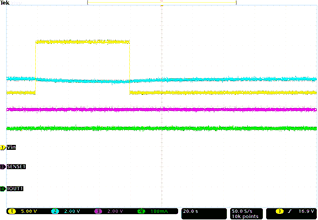Ch. 1 = V(IN) Ch. 2 = G1 Ch. 3 = SENSE1 Ch. 4 = I(OUT1)
Figure 12. Jump Start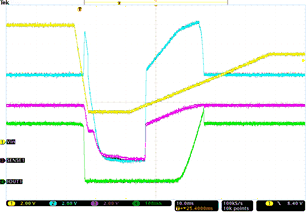Ch. 1 = V(IN) Ch. 2 = G1 Ch. 3 = SENSE1 Ch. 4 = I(OUT1)
Figure 14. Fast Power Down and Slow Power UpCh. 1 = V(IN) Ch. 2 = G1 Ch. 3 = SENSE1 Ch. 4 = I(OUT1)
Figure 16. Slow Decrease and Slow Increase of Supply Voltage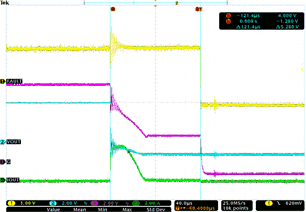Ch. 1 = FAULT Ch. 2 = SENSE1 Ch. 3 = G1 Ch. 4 = I(OUT1) LED short-to-ground on SENSE1
Figure 18. LED Hard Short Protection and One-Fails–All-Fail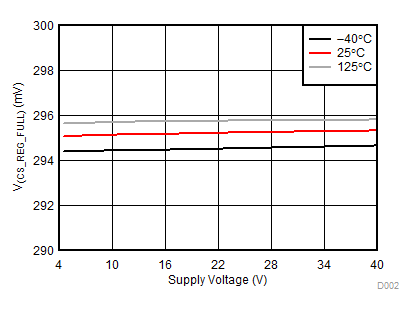V(ICTRL) = 1.8 V
Figure 3. Full-Range Current-Sense Voltage vs Supply Voltage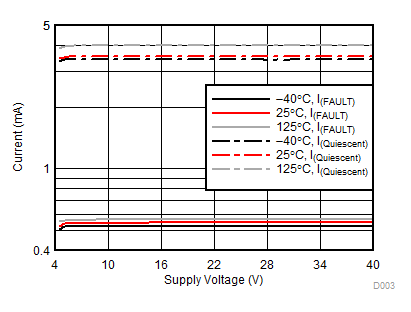Figure 5. Device Current vs Supply Voltage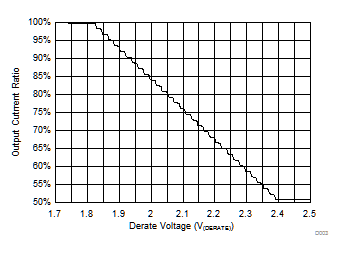V(IN) = 14 V
Figure 7. Current-Derating Profile; Output-Current Ratio vs DERATE Voltage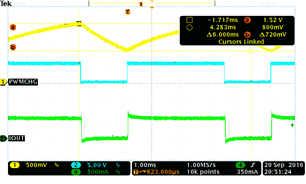Ch. 1 = PWMCHG Ch. 2 = PWMOUT Ch. 4 = I(OUT1) V(IN) = 14 V
Figure 9. PWM GeneratorCh. 1 = V(IN) Ch. 2 = G1 Ch. 3 = SENSE1 Ch. 4 = I(OUT1)
Figure 11. Transient Overvoltage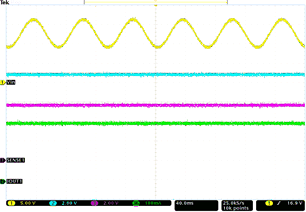Ch. 1 = V(IN) Ch. 2 = G1 Ch. 3 = SENSE1 Ch. 4 = I(OUT1) ƒ = 15 Hz
Figure 13. Superimposed Alternating Voltage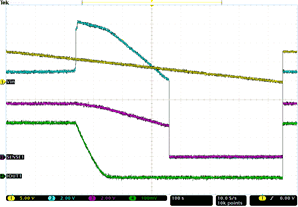Ch. 1 = V(IN) Ch. 2 = G1 Ch. 3 = SENSE1 Ch. 4 = I(OUT1)
Figure 15. Slow Decrease, Quick Increase of Supply Voltage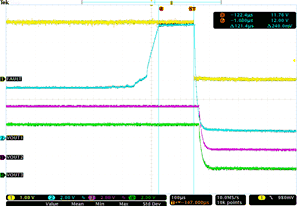Ch. 1 = FAULT Ch. 2 = SENSE1 Ch. 3 = SENSE2 Ch. 4 = SENSE3 LED open-circuit on SENSE1
Figure 17. LED Open-Circuit Protection and One-Fails–All-Fail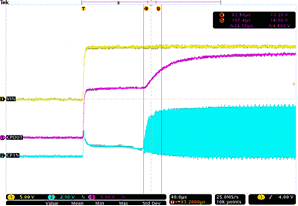Ch. 1 = V(IN) Ch. 2 = CP1N Ch. 3 = CPOUT
Figure 19. Device Start-Up Delay